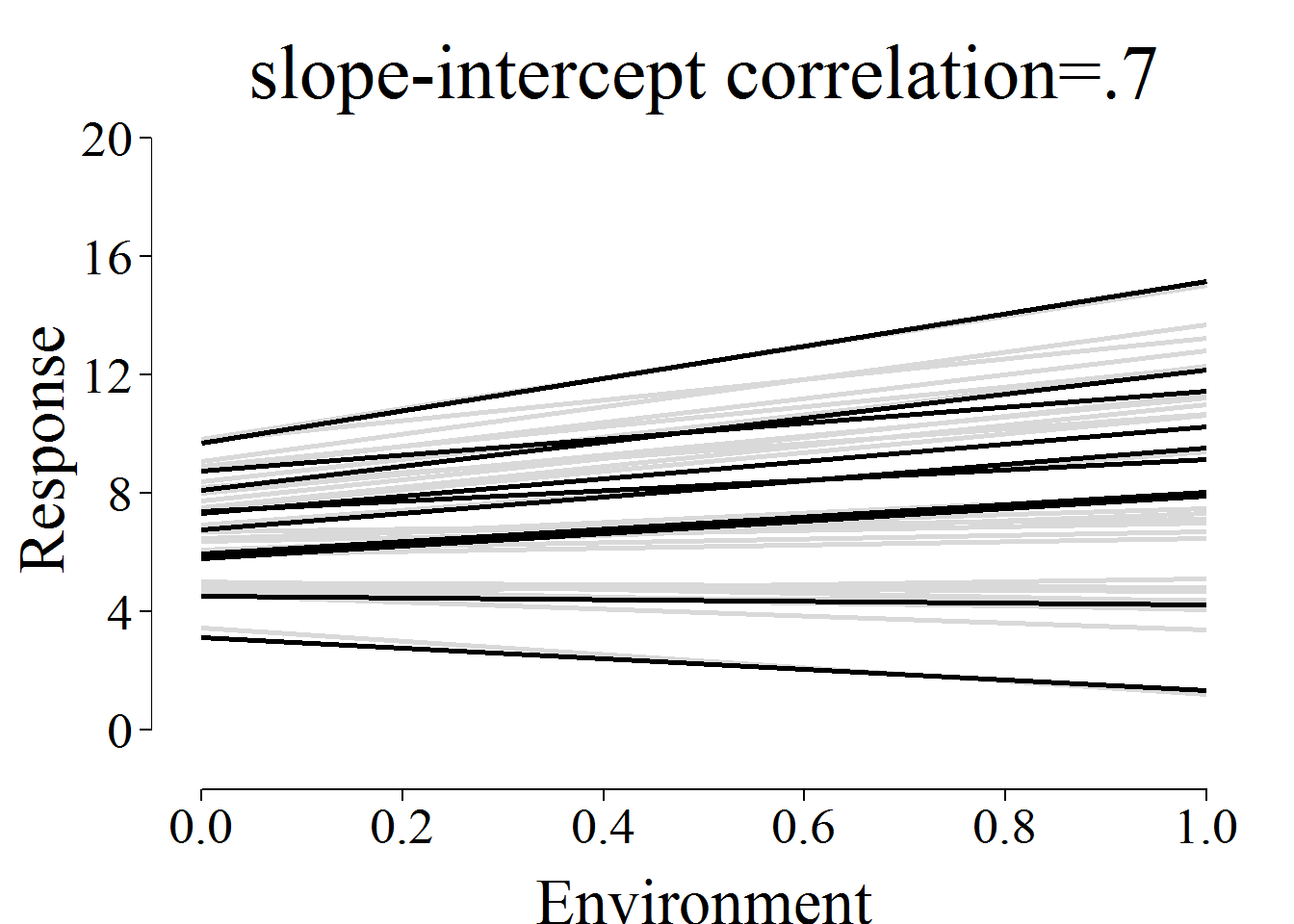I find simulations with set parameters often the best way to gain understanding of statistical power in a specific testing design. My main aim with this syntax is to generate data following a random slope, random intercept model. The response variable (‘resp’ in short) varies between individuals (‘ID’) both in intercept as its linear relation to some environmental variable (‘env’). This typically occurs if individuals differ in their mean expression of a trait but also differ in how strongly they respond to the environment (e.g. temperature).

Below syntax uses the simulate command in package lme4 (Bates et al. 2015). In contrast to info on how to simulate from a fitted mixed model (‘simulate.merMod’), the lme4 vignette only offers basic info on how to parametrize simulations for a non-fitted model (‘simulate.formula’). Below thread on the R-sig-Mixed forum ended up crucial for my understanding of how theta should be fitted.

https://stat.ethz.ch/pipermail/r-sig-mixed-models/2014q3/022378.html

Parameters that can be changed in below code are the number of ID’s scored, number of replicates per individuals, random variance in intercepts, random variance in slopes, random covariance between intercept and slope, residual variance and fixed effects.

The syntax ends with a couple of comparisons between set and achieved parameters and a plot showing variation in individual reaction norms.

require(lme4)
## Loading required package: lme4
## Loading required package: Matrix
require(ggplot2)
## Loading required package: ggplot2
require(ggthemes)
## Loading required package: ggthemes

#### Set the model the data should follow

form<-as.formula(c("~env+(env|ID)"))

Env is also fitted as a fixed effect to account for mean plasticity at the group level.

In this model there are four (co)variances fitted

1. V.ind0 = variance in reaction norm intercepts between individuals

2. V.inde = variance in reaction norm slopes between individuals

3. COVind0-ind1e = covariance between reaction norm slopes and intercepts

4. V.err0 = residual variance

#### Set the predictor variables

The number of individuals sampled:

N.ind<-40

The number of repeat obs per individual:

N.obs<-15
set.seed(25)
simdat <-data.frame(ID=factor(rep(1:N.ind,each=N.obs)),env=runif(N.ind*N.obs,0,1))

#### Set the fixed effect parameters

beta<-c(7,2)
names(beta)<-c("(Intercept)","env")

In this example the fixed effects are: Beta intercept=7 Population level slope for environment=2

#### Set the random effect structure

V.ind0 <- 3
V.inde <- 5
V.err0 <- 3
COVind0.ind1e <- 0.7*(sqrt(V.ind0*V.inde))

COVind0.ind1e with slope-intercept correlation set to 0.7

The variance covariance matrix

vcov<-matrix(c(V.ind0,COVind0.ind1e,COVind0.ind1e,V.inde), 2, 2)

Calculate theta

theta<-c((chol(vcov)/sqrt(V.err0))[1,1],
(chol(vcov)/sqrt(V.err0))[1,2],
(chol(vcov)/sqrt(V.err0))[2,2])
names(theta)<-c("ID.(Intercept)","ID.env.(Intercept)","ID.env")

#### Simulate data following above parameters

set.seed(25)
response<-simulate(form,newdata=simdat,family=gaussian,
newparams=list(theta = theta,beta = beta, sigma = sqrt(V.err0)))
simdat$resp<-as.vector(response[,1]) #### Check the simulated data against parameter settings model1<-lmer(resp~env+(env|ID),data=simdat) Do simulated betas correspond to set beta parameters? summary(model1) ## Linear mixed model fit by REML ['lmerMod'] ## Formula: resp ~ env + (env | ID) ## Data: simdat ## ## REML criterion at convergence: 2507.4 ## ## Scaled residuals: ## Min 1Q Median 3Q Max ## -2.86745 -0.64037 -0.01198 0.67059 2.70785 ## ## Random effects: ## Groups Name Variance Std.Dev. Corr ## ID (Intercept) 3.432 1.853 ## env 4.623 2.150 0.76 ## Residual 2.933 1.713 ## Number of obs: 600, groups: ID, 40 ## ## Fixed effects: ## Estimate Std. Error t value ## (Intercept) 6.7063 0.3261 20.564 ## env 1.8034 0.4216 4.277 ## ## Correlation of Fixed Effects: ## (Intr) ## env 0.328 beta ## (Intercept) env ## 7 2 Do simulated variances correspond to set variance parameters? as.data.frame(VarCorr(model1)) ## grp var1 var2 vcov sdcor ## 1 ID (Intercept) <NA> 3.432159 1.8526086 ## 2 ID env <NA> 4.623206 2.1501643 ## 3 ID (Intercept) env 3.046502 0.7647969 ## 4 Residual <NA> <NA> 2.932810 1.7125448 V.ind0 ##  3 V.inde ##  5 COVind0.ind1e ##  2.711088 V.err0 ##  3 getME(model1,"theta") ## ID.(Intercept) ID.env.(Intercept) ID.env ## 1.0817869 0.9602312 0.8089070 theta ## ID.(Intercept) ID.env.(Intercept) ID.env ## 1.0000000 0.9036961 0.9219544 #### Generate plot showing the different slopes and intercepts for all individuals For clarity 10 random individuals are shown in black, others in grey First extract random effects for plotting fittednorms<-ranef(model1)$ID[,1:2]
fittednorms[,1]<-fittednorms[,1]+getME(model1,"beta")
fittednorms[,2]<-fittednorms[,2]+getME(model1,"beta")
colnames(fittednorms)<-c("intercept","slope")

Randomly select 10 individuals to be plotted in black

set.seed(25)
rand10<-sample(1:N.ind, 10, replace = FALSE)
plot1<-ggplot(simdat,aes(env, resp))+
geom_rangeframe(data=data.frame(x=c(0, 1), y=c(0, 20)), aes(x, y))+
theme_tufte(base_size = 25)+
geom_point(colour=NA)+
geom_segment(aes(x = 0, y = intercept,xend=1, yend=intercept+slope ), data=fittednorms,colour = "gray85", size = 1)+
geom_segment(aes(x = 0, y = intercept,xend=1, yend=intercept+slope ), data=fittednorms[rand10,],colour = "black", size = 1)+
labs(x = "Environment", y="Response",title="slope-intercept correlation=.7")+
theme(axis.title.x=element_text(vjust=-1),axis.title.y=element_text(vjust=1))+
scale_x_continuous(breaks=seq(0,1,0.2))+
scale_y_continuous(breaks=seq(0,20,4))
plot1Ref
Douglas Bates, Martin Maechler, Ben Bolker, Steve Walker (2015). Fitting Linear Mixed-Effects Models Using lme4. Journal of Statistical Software, 67(1), 1-48. doi:10.18637/jss.v067.i01.